# # Generic Format

## # Purpose

The Generic Format Asset uses a specialized form of grammar language to support you in defining a structured data format you may want to ingest, output, and generally work with. The grammar language is based on regular expressions, i.e. defining data structures which can be identified using regular expressions. This includes structured text and binary formats, as well as for example JSON formats.

The editor enables you to:

1. Define the grammar of an arbitrary structured data format.
2. Test the grammar with real-life data while in edit-mode.

Once the grammar is defined and tested in the Configuration Center, it can be deployed to a Reactive Cluster as part of a Project. Remember, that a Reactive Cluster may be a single Reactive Engine running locally, e.g. on your laptop as part of the standard installation. When the Project is activated on the Cluster, the grammars which are part of this Project are read, verified and activated. The Project is then ready to ingest and output the data in the specified formats.

In addition to this, and very importantly so, the grammar also enables you to access the data within defining business logic, e.g. in Javascript and other Assets.

We will explain all of this in this document.

### # This Asset is required by:

Input Processors

Output Processors

## # Configuration

### # Name & Description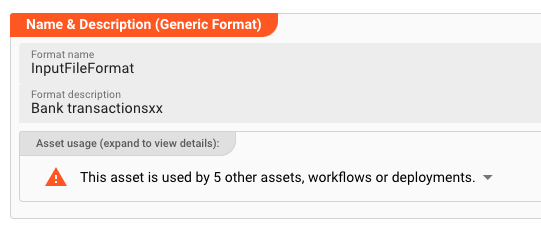`Name`: Name of the Asset. Whitespaces are not allowed in the name.

`Description`: Enter a description.

The `Asset Usage` box shows how many times this Asset is used and which parts are referencing it. Click to expand and then click to follow, if any.

### # Format Definition

Format definition describes the process of defining a format by way of the mentioned grammar language. The Configuration Center provides an editor to enter the grammar. Grammar editing is supported by just-in-time compilation of the grammar. Potential issues are highlighted. Hints can be shown by hovering over the red squiggly underlines. Hovering over property names shows clues about the purpose of the property.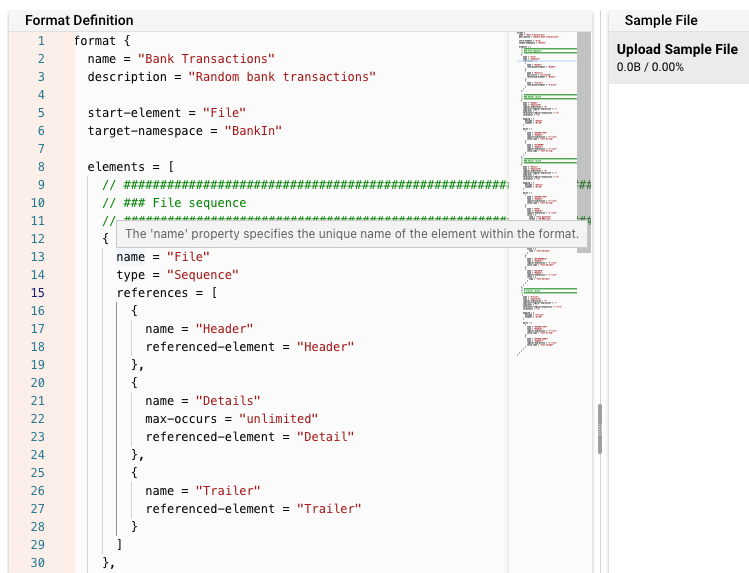The next section now describes the grammar language, which is entered in the Format Editor.

#### # Lexical Parser

This grammar which you define is internally processed by a lexical parser. This is in turn compiles a parser which is then able to ingest and/or output the defined format.

Processing event data, the lexical analyzer recognizes lexical patterns in the data. Based on the grammar, the analyzer identifies and categorizes individual elements/tokens (tokenization) by applying the regular expressions given in the grammar. An element can look like anything; it just needs to be a useful part of the structured event. Each element must have an associated regular expression which definitively identifies a specific element, however.

Looking at an example-grammar should help us to understand how it works. This example is for a simple comma-separated-format. Please note, that virtually any other structured data format can be defined using the grammar language.

``````format {
name = "Bank Transactions"                              // Name of the grammar for internal purposes.
description = "Random bank transactions"                // Description

start-element = "File"                                  //
target-namespace = "BankIn"

elements = [
// #####################################################################
// ### File sequence
// #####################################################################
{
name = "File"
type = "Sequence"
references = [
{
},
{
name = "Details"
max-occurs = "unlimited"
referenced-element = "Detail"
},
{
name = "Trailer"
referenced-element = "Trailer"
}
]
},

// #####################################################################
// #####################################################################
{
type = "Separated"
regular-expression = "H"
separator-regular-expression = ";"
separator = ";"
terminator-regular-expression = "\n"
terminator = "\n"

mapping = {
element = "BT_IN"
}

parts = [
{
name = "RECORD_TYPE"
type = "RegExpr"
regular-expression = "[^;\n]*"
value.type = "Text.String"
},
{
name = "FILENAME"
type = "RegExpr"
regular-expression = "[^;\n]*"
value.type = "Text.String"
}
]
},
// #####################################################################
// ### Detail record
// #####################################################################
{
name = "Detail"
type = "Separated"
regular-expression = "D"
separator-regular-expression = ";"
separator = ";"
terminator-regular-expression = "\n"
terminator = "\n"

mapping = {
message = "Detail"
element = "BT_IN"
}

parts = [
{
name = "RECORD_TYPE"
type = "RegExpr"
regular-expression = "[^;\n]*"
value.type = "Text.String"
},
{
name = "DATE"
type = "RegExpr"
regular-expression = "[^;\n]*"
value = {
type = "Text.DateTime"
format = "dd-MMM-uuuu"
}
},
{
name = "DESCRIPTION"
type = "RegExpr"
regular-expression = "[^;\n]*"
value = {
type = "Text.String"
}
},
{
name = "DEPOSITS"
type = "RegExpr"
regular-expression = "[^;\n]*"
value = {
type = "Text.Decimal"
}
},
{
name = "WITHDRAWALS"
type = "RegExpr"
regular-expression = "[^;\n]*"
value.type = "Text.Decimal"
},
{
name = "BALANCE"
type = "RegExpr"
regular-expression = "[^;\n]*"
value = {
type = "Text.Decimal"
}
}
]
},
// #####################################################################
// # Trailer record
// #####################################################################
{
name = "Trailer"
type = "Separated"
regular-expression = "T"
separator-regular-expression = ";"
separator = ";"
terminator-regular-expression = "\r?\n"
terminator = "\n"

mapping = {
message = "Trailer"
element = "BT_IN"
}

parts = [
{
name = "RECORD_TYPE"
type = "RegExpr"
regular-expression = "[^;\n]*"
value.type = "Text.String"
},
{
name = "RECORD_COUNT"
type = "RegExpr"
regular-expression = "[^;\n]*"
value.type = "Text.Integer"
}
]
}
]
}

``````

(The structure of the grammar language looks like JSON, but please not that while it is similar, it is NOT in JSON format.)

Looking at this example, it can be noted, that a grammar defines a hierarchical structure of elements, of which each can be of different types and structures themselves.

In the above the parser initially identifies three types of elements:

• Detail
• Trailer

Each of these elements is then again structured into "sub"-elements which represent the individual fields. It's a fairly simple format.

On ingestion of the event message and parsing of the data, the result is written to an internal data format. This now structured data can be accessed and manipulated throughout the configured processing stages of the Project.

The whole process works in reverse, when outputting data using the defined grammar.

#### # Language syntax

Let's understand the structure and elements which can be used to define your grammar.

##### # Format

The top node of any grammar is `format`. Everything else is defined underneath it. It represents the bracket of every grammar.

Syntax Diagram: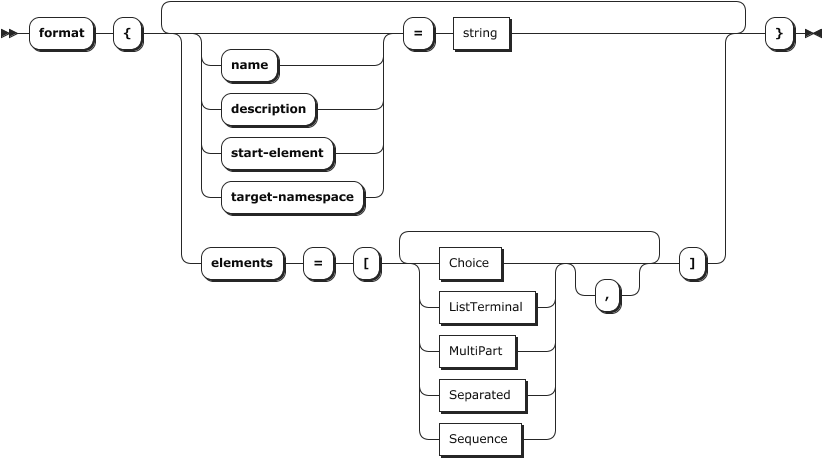Properties:

Attribute Type Description Optional Default
name string Name of the format
description string Description of the format x
start-element string Name of the element which starts parsing. See`elements` below.
target-namespace string Internal name of the target namespace. Must be unique across all formats
elements [] Array of the typesChoice, ListTerminal, MultiPart, Separated, Sequence Empty array

Example:

``````format {
name = "Bank Transactions"
description = "Random bank transactions"

start-element = "File"
target-namespace = "BankIn"

elements = [
// #####################################################################
// ### File sequence
// #####################################################################
{
name = "File"
type = "Sequence"
references = [
{
},
{
name = "Details"
max-occurs = "unlimited"
referenced-element = "Detail"
},
{
name = "Trailer"
referenced-element = "Trailer"
}
]
},
...
``````
##### # Elements

`Elements` are part of the format definition. The following list of elements exist:

Logical Elements

Terminal Elements

###### # Choice

A `Choice` element is a "logical selector" between a number of possible next elements for the parser. However, only one of the paths is valid for the given message data. In other words, this element both limits and opens which elements are possible to be next in the parsing process.

This type of element does not parse data. It serves to decide which structure should be used for forward parsing. Use this if there are different options of what the next strcuture could be.

Used in:

Syntax Diagram: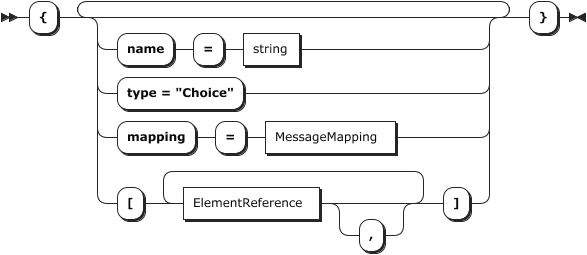Properties:

Attribute Type Description Optional Default
name string Name of the element
type "Choice" Must be "Choice"
mapping MessageMapping Specifies if the choice shall be mapped into a separate message X
references ElementReference[] Array of referenced alternatives X Empty array

Example:

``````...
// #####################################################################
// ### Detail choice
// #####################################################################
{
name = "DetailChoice"
type = "Choice"
references = [
{
name = "Carment_19_2"
referenced-element = "DETAIL_19_2"        // Next element must be either DETAIL_19_2 as defined below ...
},
{
name = "Carment_19_3"
referenced-element = "DETAIL_19_3"        // ... or this element
}
]
},
// #####################################################################
// ### Detail Carmen 19.2 record
// #####################################################################
{
name = "DETAIL_19_2                         // Is being referenced from above
type = "Separated"
regular-expression = "XYZ(;([^;\n]*)){60}\n"
separator-regular-expression = ";"
separator = ";"
terminator-regular-expression = "\n"
terminator = "\n"

...
``````
###### # Sequence

Representation of a logically ordered set of element types. For each element defined in `references` there must be `0..n` occurrences. Like the Choice element, this element does not parse data, but further defines rules for the structure of the file.

Used in:

Syntax Diagram: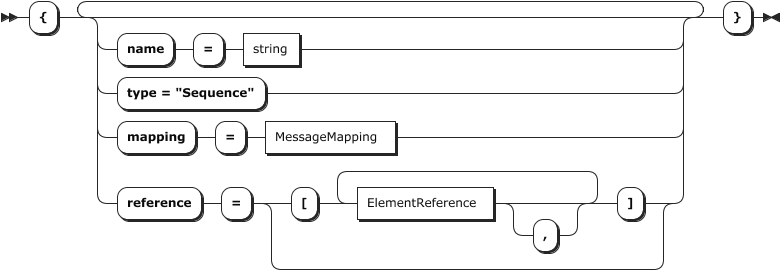Properties:

Attribute Type Description Optional Default
name string Name of the element
type "Sequence" Specifies the type of the element
mapping MessageMapping Specifies if the element shall be mapped into a separate message. This will create a whole new message in layline.io. X
references ElementReference[] Array of sub-elements X Empty array

Example: In this example the file structure is defined as such that it

2. must be followed by `0..n` records of type Detail
3. must be followed by one record of type Trailer
``````...
elements = [
// #####################################################################
// ### File sequence
// #####################################################################
{
name = "File"
type = "Sequence"
references = [
{
},
{
name = "Details"
min-occurs = 0
max-occurs = "unlimited"
},
{
name = "Trailer"
referenced-element = "Trailer"          // Last element must be defined element "Trailer"
}
]
},
...
]

``````
###### # ListTerminal

Element which detects a list of elements where

1. the list is terminated at the end of hte list
2. the individual elements in the list are separated by a separator

Use this element to work with formats which

1. have records with an arbitrary number of fields in a record
2. each field is of the same type

Example data:

``````Id,Name,Surname,Street,City                      // First line contains column headers --> this is the ListTerminal
10,John,Doe,MyStreet,MyCity                      // Data records contain the actual data
20,Lara,Croft,Tombstone St.,Death Valley
...
``````

Used in:

Syntax Diagram: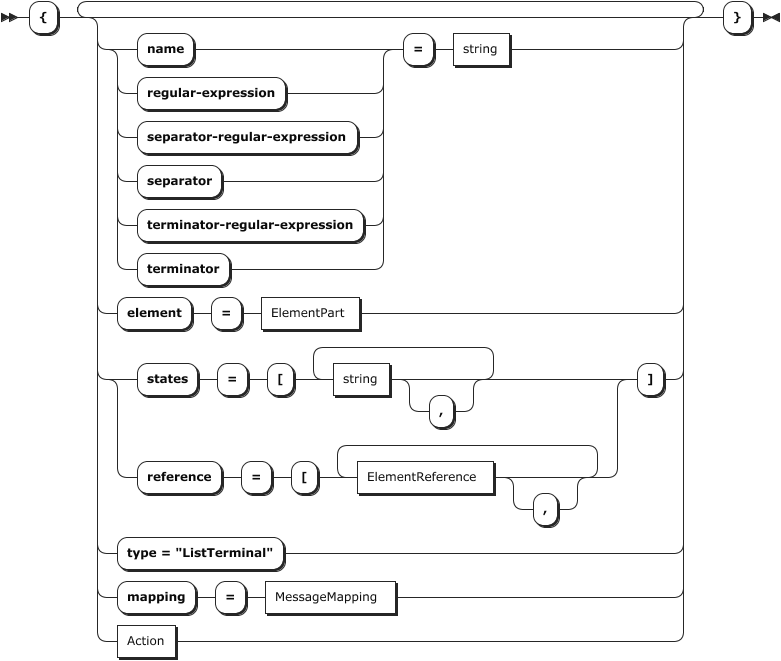Properties:

Attribute Type Description Optional Default
name string Name of the element
type "ListTerminal" Specifies the type of the element
mapping MessageMapping Specifies if the element shall be mapped into a separate message X
regular-expression string Regular expression used to detect the Separated element
states string [] List of state names in which the Separated element is detected X Empty array
action Action Action that is executed, when the Separated element is detected X No action
separator-regular-expression string Regular expression used to detect the field separator X ","
separator string Separator to use when the element is written X ","
terminator-regular-expression string Regular expression used to detect the terminator of the element X "\r?\n"
terminator string Terminator to use when the element is written X "\n"
element ElementPart Element part which describes the elements in the list. One part defines alle elements.
references ElementReference[] Array of referenced elements behind the list X Empty array

Example: This example works for the example data above. At first we detect and parse the Header columns, and following this then all Detail records.

``````

...
elements = [
// File sequence
{
name = "File"
type = "Sequence"
references = [
{
type = "Reference"
},
{
name = "Details"
type = "Reference"
max-occurs = "unlimited"
referenced-element = "Detail"
}
]
},
{
name = "Header"                           // Element name
type = "ListTerminal"                     // The fixed type name "ListTerminal"
regular-expression = ".*\n"               // Matching data
action = "pushState(DETAIL_STATE)"        // This element, switches the parser state to "DETAIL_STATE"

separator-regular-expression = ","        // regex to find the separator
separator = ","
terminator-regular-expression = "\r?\n"   // regex to find the terminator
terminator = "\n"

mapping = {
message = "Header"                    // message will be mapped as a message of type "Header"
element = "XYZ"
}

element = {                               // This example defines an element of type "RegExpr"
name = "RecordId"
type = "RegExpr"
regular-expression = "[^,\r\n]*"      // Regular expresssion to find the element
value.type = "Text.String"            // Type of ALL found elements. Can only be one type for all.
}
},
{
name = "Detail"
type = "ListTerminal"
regular-expression = ".*\n"
states = [
"DETAIL_STATE"                        // Parser state must be in "DETAIL_STATE" for this element to be parsed (see above).
]

separator-regular-expression = ","
separator = ","
terminator-regular-expression = "\r?\n"
terminator = "\n"

mapping = {
message = "Detail"
element = "OMS"
}

element = {
name = "RecordId"
type = "RegExpr"
regular-expression = "[^,\r\n]*"
value.type = "Text.String"
}
}
]
...
``````
###### # MultiPart

Element which detects a record where

1. the end of the record can be identified by regular expression,
2. the record contains a number of (sub-)elements, or parts,
3. the sub-elements can be defined as element-parts.

Use this if you

1. have a record with individual (sub-)elements,
2. each sub-element could be either of type Fixed, Calculated, or RegExpr.

Used in:

Syntax Diagram: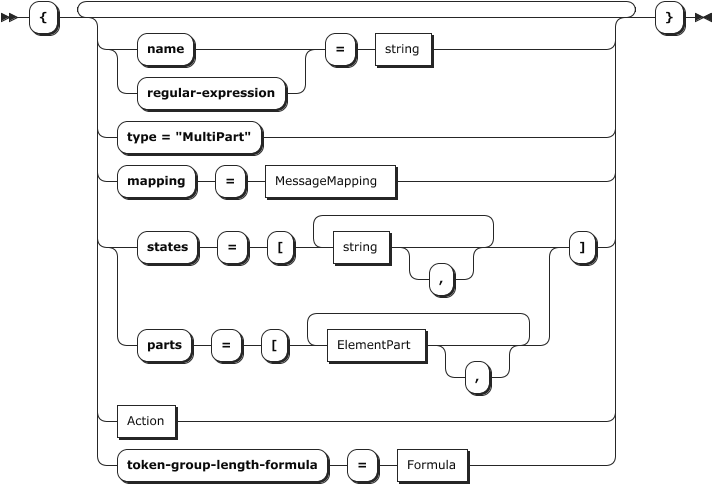Properties:

Attribute Type Description Optional Default
name string Name of the element
type "MultiPart" Specifies the type of the element
mapping MessageMapping Specifies if the element shall be mapped into a separate message X
regular-expression string Regular expression used to detect the separated element
states string [] List of state names in which the Separated element is detected X Empty array
action Action Action that is executed, when the Separated element is detected X No action
parts ElementPart[] Array of element parts within the element X Empty array
token-group-length-formula Formula Formula used to calculate the length of the entire token group X No formula

Example:

``````...
{
name = "Person"                   // Element name
type = "MultiPart"                // Of fixed type "MultiPart"
regular-expression = ".*\n"       // Regular expression to detect this element. Terminated by by new line

mapping = {
message = "Person"
element = "Data"
}

parts = [                         // Array of elements which make up the MultiPart element
{
name = "RecordId"             // The first element is the Record ID
type = "Fixed"                // of fixed length
length = 3                    // Length is 3
justification = "left"        // left justified
value.type = "Text.String"    // data type is "String"
},
{
name = "Surname"
type = "Fixed"
length = 20
justification = "left"
value = {
type = "Text.String"
encoding = "utf-8"
}
},

...

{
name = "EOL"
type = "RegExpr"
regular-expression = "\r?\n*"
value.type = "Text.String"
}
]
}
...

``````
###### # Separated

Terminal element which is detected by a regular expression and consists of multiple parts separated by a separator and terminated by a terminator. These are typically records containing fields which are separated by a delimiter character (e.g. `,` or `;`). The complete record may be terminated by another character (e.g. `\n` or `\r\n` as record separator). Because the lexical analyzer has no length information for the record, the regular expression specified as pattern has to match the full record including the record separator.

Used in:

Syntax Diagram: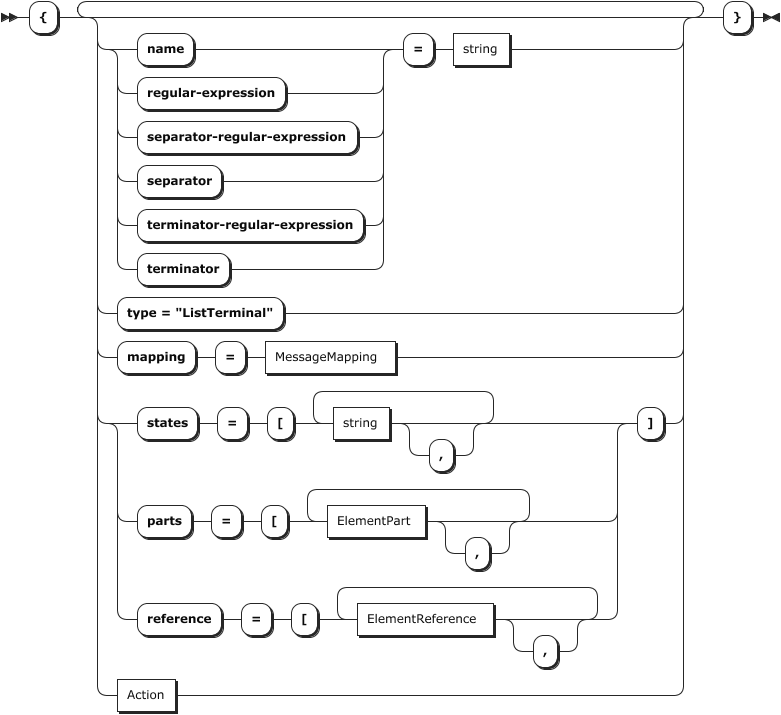Properties:

Attribute Type Description Optional Default
name string Name of the element
type "Separated" Specifies the type of the element
mapping MessageMapping Specifies if the element shall be mapped into a separate message. This will create a whole new message in layline.io. X
regular-expression string Regular expression used to detect the Separated element.
Example:
10.*\n. The record must start with the string "10" followed by zero or more characters, and must end with the new line character.
states string [] List of state names in which the Separated element is detected X Empty array
action Action Action that is executed, when the Separated element is detected X No action
separator-regular-expression string Regular expression used to detect the field separator and splitting the record up in its field components. X ","
separator string Separator to use when the element is output X ","
terminator-regular-expression string Regular expression used to detect the terminator of the element. E.g.`\r\n` as CRLF. X "\r?\n"
terminator string Terminator to use when the element is output X "\n"
parts ElementPart[] Array of element parts within the element, e.g. the fields. X Empty array
references ElementReference[] Array of sub-elements X Empty array

Example:

``````...
//==================
//==================
{
type = "Separated"
regular-expression = "10.*\n"
separator-regular-expression = ","
separator = ","
terminator-regular-expression = "\r?\n"
terminator = "\n"

mapping = {
element = "CSV"
}

parts = [
{
name = "RecordId"
type = "RegExpr"
regular-expression = "[^,\r\n]*"
value.type = "Text.String"
},
{
name = "Sender"
type = "RegExpr"
regular-expression = "[^,\r\n]*"
value = {
type = "Text.String"
encoding = "utf-8"
}
},
...
{
name = "ZoneOffset"
type = "RegExpr"
regular-expression = "[^,\r\n]*"
value = {
type = "Text.String"
encoding = "utf-8"
}
}
]
},
...

``````
##### # Element Parts

The following list of elements exist:

Used in:

###### # Calculated

A terminal element part which has a length calculated from some data in the input data stream.

Syntax Diagram: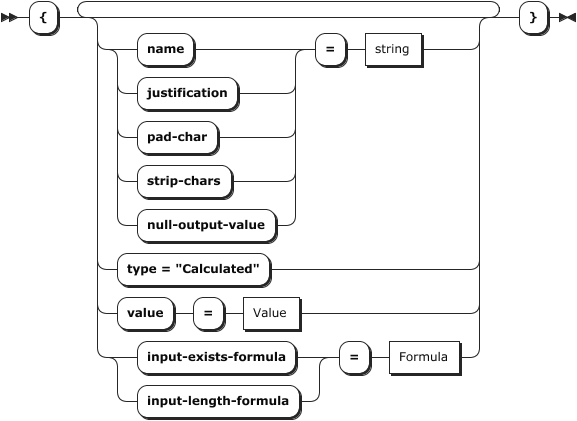Properties:

Attribute Type Description Optional Default
name string Name of the element
type "Calculated" Specifies the type of the element part
value Value Value of the element
input-exists-formula Formula Boolean formula that specifies if the element exists. The formula can reference another field in its calculation.
Example: `bitIsSet(other_field,31)"` --> the field exists if other_field has bit set at position 31.
X true
input-length-formula Formula Fiexed value or formula that provides the length of the data
justification string Justification of the value data (left or right) X left
pad-char string Padding character used when the value is output X " " (Space)
strip-chars string String containing all characters that shall be striped when the value is input X " " (Space)
null-output-value string Value that shall be used as output when the element part is null X not set

Example:

``````...
{
name = "Bitmap3"
type = "Calculated"
input-exists-formula = "bitIsSet(Bitmap1,31)"
input-length-formula = "8"
value = {
type = "Text.Long"
base = 16
}
},
...

``````
###### # Fixed

A terminal element part with a fixed length.

Syntax Diagram: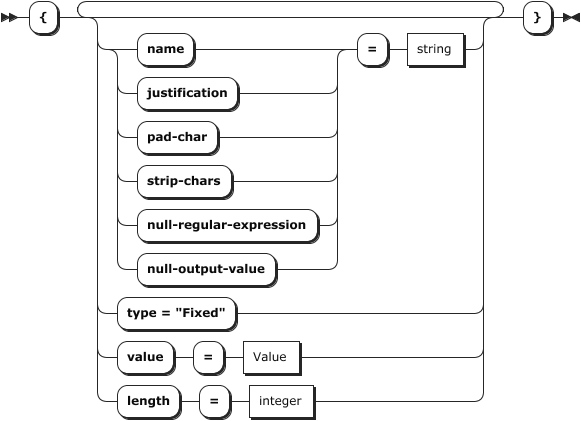Properties:

Attribute Type Description Optional Default
name string Name of the element
type "Fixed" Specifies the type of the element part
value Value Value of the element
length integer Length of the fixed element
justification string Justification of the value data (left or right) X left
pad-char string Padding character used when the value is written X " " (Space)
strip-chars string String containing all characters that shall be striped when the value is read X " " (Space)
null-regular-expression string If the value matches this regular expression it is handled as null value X not set
null-output-value string Value that shall be used as output when the element part is null X not set

Example:

``````...
{
name = "CREATE_DATE"
type = "Fixed"
length = 14
justification = "left"
null-regular-expression = "[ \t]*"
value = {
type = "Text.DateTime"
format = "uuuuMMddHHmmss"
null-input-value = "1970-01-01T00:00:00Z"
}
},
...

``````
###### # RegExpr

A terminal element part which is detected using a regular expression.

Syntax Diagram: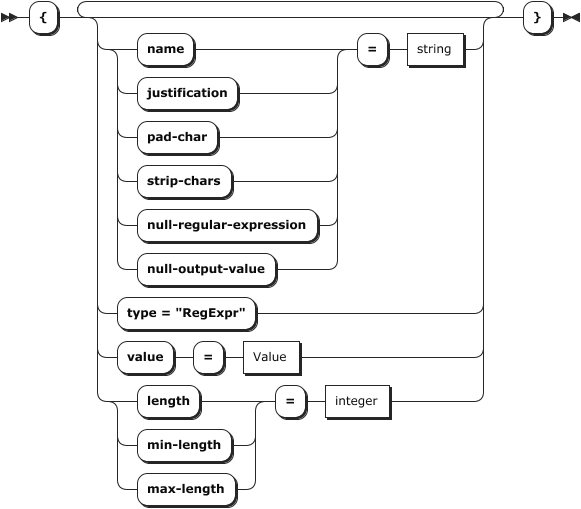Properties:

Attribute Type Description Optional Default
name string Name of the element
type "RegExpr" Specifies the type of the element part
value Value Value of the element
regular-expression string Regular expression used to detect the value in the input stream
justification string Justification of the value data (left or right) X left
pad-char string Padding character used when the value is output X " " (Space)
strip-chars string String containing all characters that shall be striped when the value is input X "" (Empty)
min-length integer Minimum length on the output side X 0
max-length integer Maximum length on the output side X unlimited
null-regular-expression string If the value matches this regular expression it is handled as null value X not set
null-output-value string Value that shall be used as output when the element part is null X not set

Example:

``````...
{
name = "RECORD_TYPE"
type = "RegExpr"
regular-expression = "[^;\n]*"
value.type = "Text.Integer"
},
...

``````
##### # Message Mapping

The MessageMapping specifies, how the current element is mapped into a layline.io message.

Used in:

Syntax Diagram: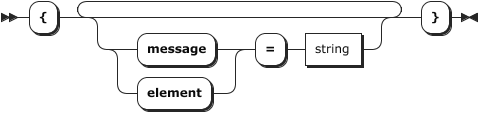Properties:

Attribute Type Description Optional Default
message string Name of the created layline.io message
element string Access path of the target element of the mapping. This is the name of the path which is used within layline.io to access the element, e.g. in a script, in field mapping, etc. X

Example:

``````...
{
name = "DETAIL_Carmen_19_3"
type = "Separated"
regular-expression = "GSM(;([^;\n]*)){61}\n"
separator-regular-expression = ";"
separator = ";"
terminator-regular-expression = "\n"
terminator = "\n"

mapping = {
message = "Detail"
element = "D1_GSM"
}
...

``````
##### # Element Reference

An ElementReference is used to reference another element from a Choice, ListTerminal, Sequence, or Sequence. In the example below a `Sequence` is defined as a number of references to elements which are defined in another place within the grammer. E.g. the Sequence is a sequence of Header, Details, and a Footer element.

Used in:

Syntax Diagram: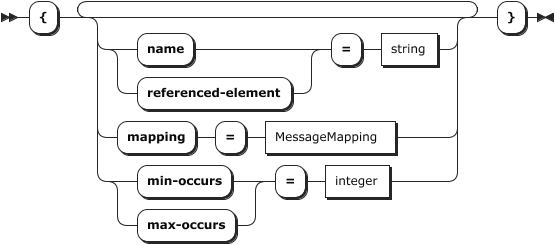Properties:

Attribute Type Description Optional Default
name string Name of the reference
mapping MessageMapping Specifies if the reference shall be mapped into a separate message X
referenced-element string Name of the referenced element. This is an element which was defined by you elsewhere in the grammar.
min-occurs integer Minimum number of occurrences X 1
max-occurs integer/"unlimited" Maximum number of occurrences X 1

Example:

``````...
elements = [
// #####################################################################
// ### File sequence
// #####################################################################
{
name = "File"
type = "Sequence"                             // Example for "Sequence"
references = [
{
referenced-element = "Header"           // The actually referenced element is also called "Header". It must be defined elsewhere in the grammar.
},
{
name = "Details"                        // Reference name is "Details"
min-occurs = 0                          // Must occur zero times --> so it is optional
max-occurs = "unlimited"                // Can occur an unlimited number of times
referenced-element = "DetailChoice"     // The actually referenced element is called "DetailChoice". It must be defined elsewhere in the grammar.
},
{
name = "Trailer"                       // Reference name is "Trailer"
referenced-element = "Trailer"         // The actually referenced element is also called "Trailer". It must be defined elsewhere in the grammar.
}
]
},

// #####################################################################
// #####################################################################
{
name = "Header  "                           // This is the element being referenced above.
type = "MultiPart"
regular-expression = "10"

mapping = {
element = "D2_AID"
}

parts = [
...

``````
##### # Value

The following list of elements exist:

Syntax Diagram: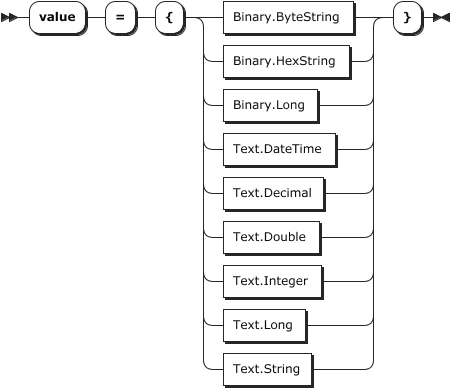###### # Binary.ByteString

A binary ByteString value. The bytes from the input will be directly mapped into a ByteString value in the message

Syntax Diagram: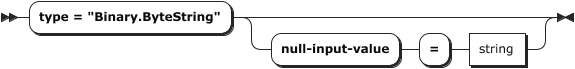Properties:

Attribute Type Description Optional Default
type "Binary.ByteString" Specifies the type of value
null-input-value string Value that will be used when the element part is classified as null X not set

Example:

``````...
{
name = "F65_ReservedForIsoUse"
type = "Calculated"
input-exists-formula = "bitIsSet(Bitmap3,31)"
input-length-formula = "8"
value = {
type = "Binary.ByteString"
}
},
...

``````
###### # Binary.HexString

A value where the binary ByteString value is encoded as hexadecimal string.

Syntax Diagram: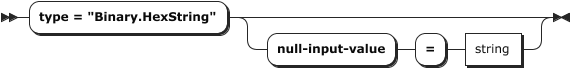Properties:

Attribute Type Description Optional Default
type "Binary.HexString" Specifies the type of value
null-input-value string Value that will be used when the element part is classified as null X not set

Example:

``````...
{
name = "F65_ReservedForIsoUse"
type = "Calculated"
input-exists-formula = "bitIsSet(Bitmap3,31)"
input-length-formula = "8"
value = {
type = "Binary.HexString"
}
},
...

``````
###### # Binary.Long

A value where the binary ByteString value is encoded as hexadecimal string.

Syntax Diagram: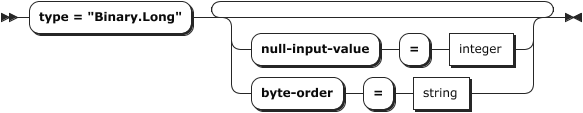Properties:

Attribute Type Description Optional Default
type "Binary.Long" Specifies the type of value
null-input-value integer Value that will be used when the element part is classified as null X not set
byte-order string Byte order of the data "BIG_ENDIAN", "BIG-ENDIAN", "BIGENDIAN", "MSBF", "LITTLE_ENDIAN", "LITTLE-ENDIAN", "LITTLEENDIAN", "LSBF" X "BIG-ENDIAN"

Example:

``````...
{
name = "MessageLength"
type = "Fixed"
length = 2
value = {
type = "Binary.Long"
}
},
...

``````
###### # Text.DateTime

A DateTime value in text format.

Syntax Diagram: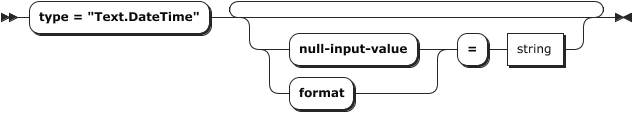Properties:

Attribute Type Description Optional Default
type "Text.DateTime" Specifies the type of value
format string Date/Time format (opens new window) X "yyyyMMddHHmmss"
null-input-value string Value that will be used when the element part is classified as null. Format like "2011-12-03T10:15:30+01:00" X not set

Example:

``````...
{
name = "Created"
type = "RegExpr"
regular-expression = "[^,\r\n]*"
value = {
type = "Text.DateTime"
format = "uuuuMMddHHmmss"
}
},
...

``````
###### # Text.Decimal

A Decimal value in text format.

Syntax Diagram: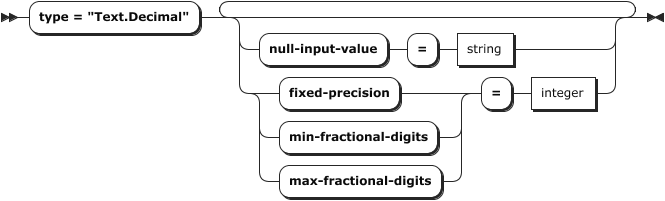Properties:

Attribute Type Description Optional Default
type "Text.Decimal" Specifies the type of value
fixed-precision integer Use a fixed precision X not set
min-fractional-digits integer Minimum number of fractional digits on the output side X 0
max-fractional-digits integer Maximum number of fractional digits on the output side X 6
null-input-value string Value that will be used when the element part is classified as null as standard decimal string. X not set

Example:

``````...
{
name = "TOTAL_CHARGE"
type = "RegExpr"
regular-expression = "[^;\n]*"
value = {
type = "Text.Decimal"
}
},
...

``````
###### # Text.Double

A Double value in text format.

Syntax Diagram: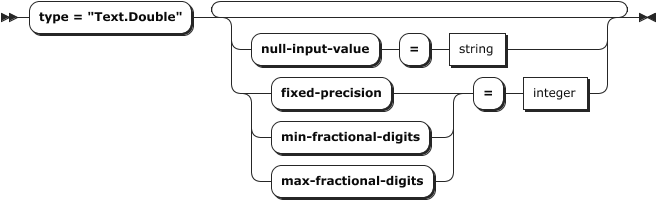Properties:

Attribute Type Description Optional Default
type "Text.Double" Specifies the type of value
fixed-precision integer Use a fixed precision X not set
min-fractional-digits integer Minimum number of fractional digits on the output side X 0
max-fractional-digits integer Maximum number of fractional digits on the output side X 6
null-input-value string Value that will be used when the element part is classified as null as standard decimal string. X not set

Example:

``````...
{
name = "X"
type = "RegExpr"
regular-expression = "[^ \r\n]*"
value = {
type = "Text.Double"
}
},...

``````
###### # Text.Integer

A 32-bit whole number value in text format.

Syntax Diagram: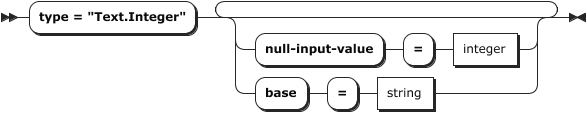Properties:

Attribute Type Description Optional Default
type "Text.Integer" Specifies the type of value
base integer Base of encoded text value (8, 10, 16). X 10
null-input-value integer Value that will be used when the element part is classified as null. X not set

Example:

``````...
{
name = "RECORD_SIZE"
type = "Fixed"
length = 4
justification = "right"
strip-chars = " "
null-regular-expression = "[ \t]*"
value {
type = "Text.Integer"
null-input-value = 0
}
}
,...

``````
###### # Text.Long

A 64-bit long value in text format.

Syntax Diagram: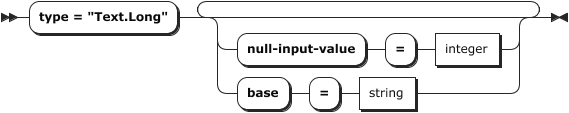Properties:

Attribute Type Description Optional Default
type "Text.Long" Specifies the type of value
base integer Base of encoded text value (8, 10, 16). X 10
null-input-value integer Value that will be used when the element part is classified as null. X not set

Example:

``````...
{
name = "Age"
type = "Fixed"
length = 3
justification = "left"
value = {
type = "Text.Long"
}
},
...

``````
###### # Text.String

A String value.

Syntax Diagram: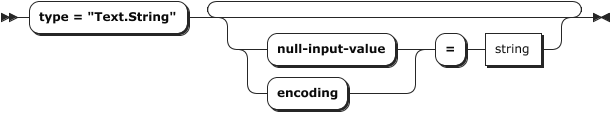Properties:

Attribute Type Description Optional Default
type "Text.String" Specifies the type of value
encoding string Used string encoding (standard Java character set names (opens new window). X "UTF-8"
null-input-value integer Value that will be used when the element part is classified as null. X not set

Example:

``````...
{
name = "F25_PointOfServiceConditionCode"
type = "Calculated"
input-exists-formula = "bitIsSet(Bitmap1,7)"
input-length-formula = "2"
value = {
type = "Text.String"
}
},
...

``````
##### # Action

An important feature of the lexical analyser is the support of different analyser states. These states are needed if not all lexical entities of a file can be described with one set of unique regular expressions. When a special record is recognized the lexical analyser can switch to another state to assign a record recognized by a regular expression to a different record type. The following action can be performed when a state should change:

• `popState`: The lexical analyser switches back to the last state that was pushed on the stack
• `pushState(state name(s))`: Tells the lexical analyser to push the current analyser state(s) on the stack and switch to the specified state. It is allowed to push multiple states within one action. It is possible to push multiple states.
• `changeState(state name)`: The lexical analyser switches to the specified state when this record is recognized.

Used in:

Syntax Diagram: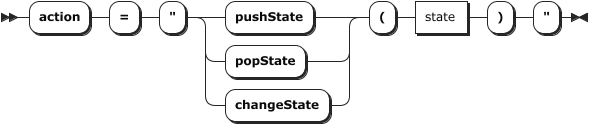##### # Formula

The `Formula` is based on layline.io's internal `QuickScript` language. Please check the QuickScript Language Reference for more info.

Used in:

Syntax Diagram: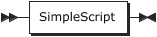Example:

``````...
{
name = "Bitmap3"
type = "Calculated"
input-exists-formula = "bitIsSet(Bitmap1,31)"
input-length-formula = "8"
value = {
type = "Text.Long"
base = 16
}
},
...

``````
##### # Data types
###### # string

Syntax Diagram: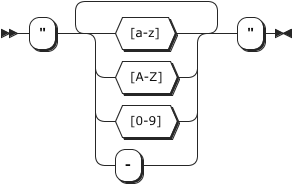###### # integer

Syntax Diagram: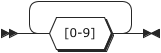###### # decimal

Immutable, arbitrary-precision signed decimal numbers. A BigDecimal consists of an arbitrary precision integer unscaled value and a 32-bit integer scale. If zero or positive, the scale is the number of digits to the right of the decimal point. If negative, the unscaled value of the number is multiplied by ten to the power of the negation of the scale. The value of the number represented by the BigDecimal is therefore (unscaledValue × 10^scale).

This data type is based on Java's BigDecimal data type from which the description is also taken. See here (opens new window) for further reference.

Syntax Diagram:###### # comment

Syntax Diagram: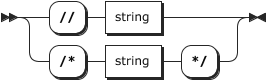#### # Sample File Testing

##### # Matching grammar and data

The UI provides the capability to not only define the grammar for your format, but readily test whether it actually works. All of this without having to go through the round-trip of deploying a whole Project to a cluster, running it, only to find out that you made a configuration mistake in the grammar.

Next to the Format-Definition Editor is the Sample-File Viewer. This is where you can upload a sample file with real-life data to see whether your grammar matches your data.

In the image below we have loaded a small sample file for our example grammar which we have introduced at the beginning of this description. It has a Header, two Detail records, and a Trailer record.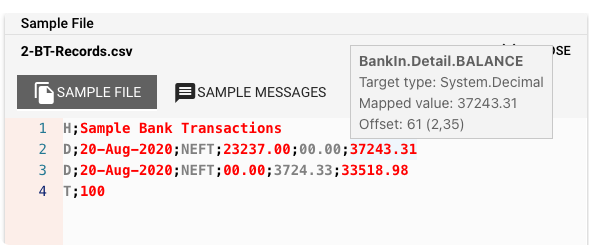If your file matches the grammar, you will see that the editor has split the data up in fields of alternating color (black and red). Hovering the mouse over a field will show a popup with information about the field (see "BankIn.Detail.Balance" in the image above.)

If you select the `Sample Messages` tab, you should see your data in a structured format: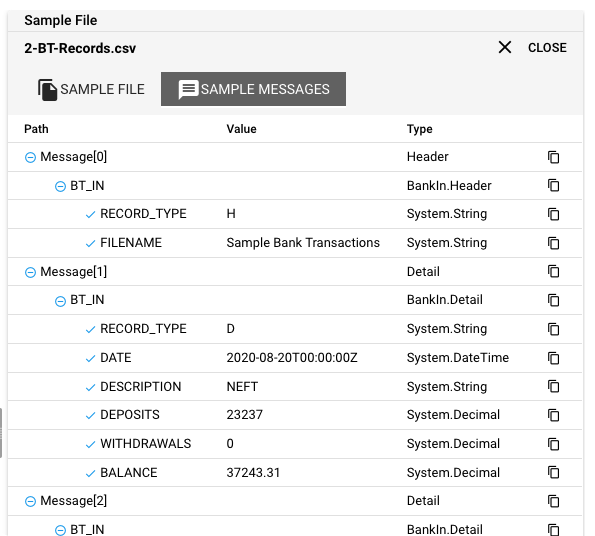To copy a part of the structure, click the copy button next to it.

There are two caveats with this approach:

1. Only works with ASCII data. Binary data viewing will be available shortly.
2. Only works with files. In other words: If you are working with streaming data / real-time data you have to put your data structures in a file first, to test whether data matches grammar.
##### # In the case of error

There are a number of reasons why the grammar may not match the data. Let's take a look what they are and how to remedy them:

1. Grammar contains red squiggly lines under items: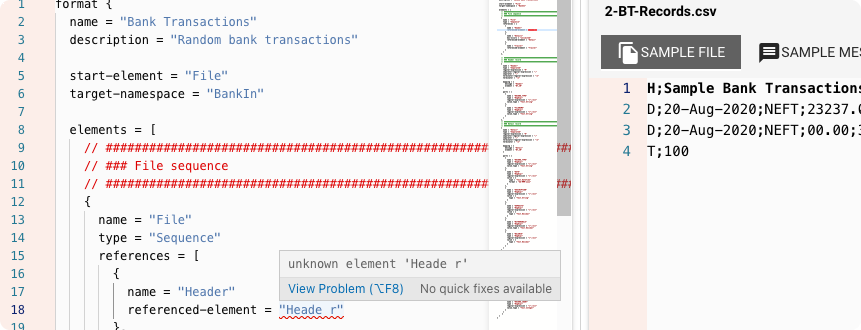This indicates a problem with the grammar. Hover over the marked item and check the popup which should show the cause of the problem.

1. Grammar does not match sample data

In this case the grammar is probably not fully configured or there is some other problem. A typical problem is that the data can be partially parsed, while some of the fields are not correctly recognized. In this case it should be fairly easy to allocate the problem within the grammar.

I tried everything and still need help!

Don't be frustrated in case you run into issues configuring more complex formats! Help is just around the corner. Please just get in touch (opens new window) with us, and we WILL help you to set up your format!

### # Internal

Input Processors

Output Processors

## # Information missing in the documentation?

Can't find what you are looking for?

Please note, that the creation of the online documentation is Work-In-Progress. It is constantly being updated. Should you have questions or suggestions, please don't hesitate to contact us at support@layline.io.

Last Updated: 4/28/2022, 3:40:43 PM